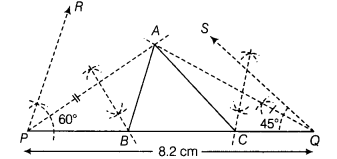# Construct a ∆ABC whose perimeter is 8.2 cm and the base angles are 45° and 60°

Construct a ∆ABC whose perimeter is 8.2 cm and the base angles are 45° and 60°.

Steps of construction
(i) First, draw PQ = 8.2 cm.
(ii) At point P, construct ∠RPQ = 60° and at point Q,
construct ∠SQP = 45°.
(iii) Draw bisector of ∠RPQ and∠SQP, which intersect each other at point A.
(iv) Join AP and AQ.
(v) Draw perpendicular bisector of AP which intersects PQ at point B and also draw perpendicular bisector of AQ which intersects PQ at point C.(vi) Join AB and AC Thus, we get the required ∆ABC.